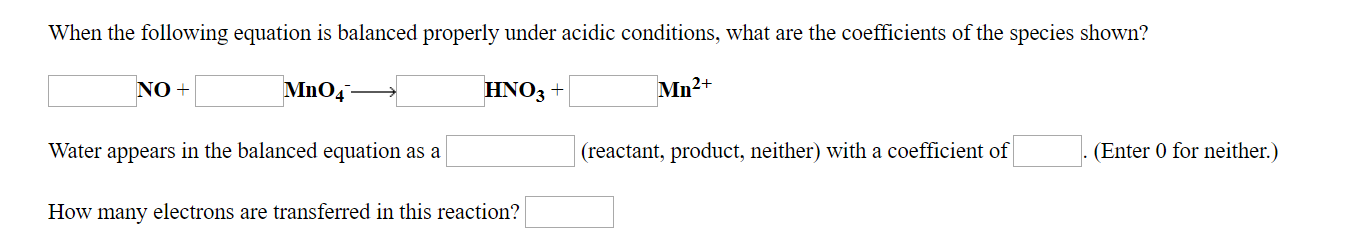When the following equation is balanced properly under acidic conditions, what are the coefficients of the species shown?Mn2+HNO3+NO+(reactant, product, neither) with a coefficient ofEnter 0 for neither.)Water appears in the balanced equation as aHow many electrons are transferred in this reaction?

Questionhelp_outlineImage TranscriptioncloseWhen the following equation is balanced properly under acidic conditions, what are the coefficients of the species shown? Mn2+ HNO3+ NO+ (reactant, product, neither) with a coefficient ofEnter 0 for neither.) Water appears in the balanced equation as a How many electrons are transferred in this reaction? fullscreen
Step 1

For balancing a redox reaction, certain steps are followed.

STEP 1 : Writing the skeletal equation and calculating the oxidation number on all atoms.

For calculating oxidation number, certain rules need to be followed:

Oxidation no. of O in almost all of its compounds is -2.

Oxidation no. of H in most of its compounds is +1.

The sum of oxidation numbers of all atoms in a species is equal to the total charge on the species.

NO:

Let oxidation no. of N be x:      x-2 = 0     or x = +2

MNO4-:

Let oxidation no. of Mn be y           y + 4(-2)=-1    or         y = +7

HNO3       : Let oxidation number of N be z

1+z+3(-2)=0     or z = +5

Step 2

STEP 2 : Separating the equation into two half reactions-oxidation (increase in oxidation no.)  half reaction and reduction (decrease in oxidation no.) half reaction.

Step 3

STEP 3: Balancing atoms other than O and H in the two half reactions:

I...

Want to see the full answer?

See Solution

Want to see this answer and more?

Our solutions are written by experts, many with advanced degrees, and available 24/7

See Solution
Tagged in

Chemistry